Find solution of equations- Statement given

Chapter 4 Class 12 Determinants
Concept wise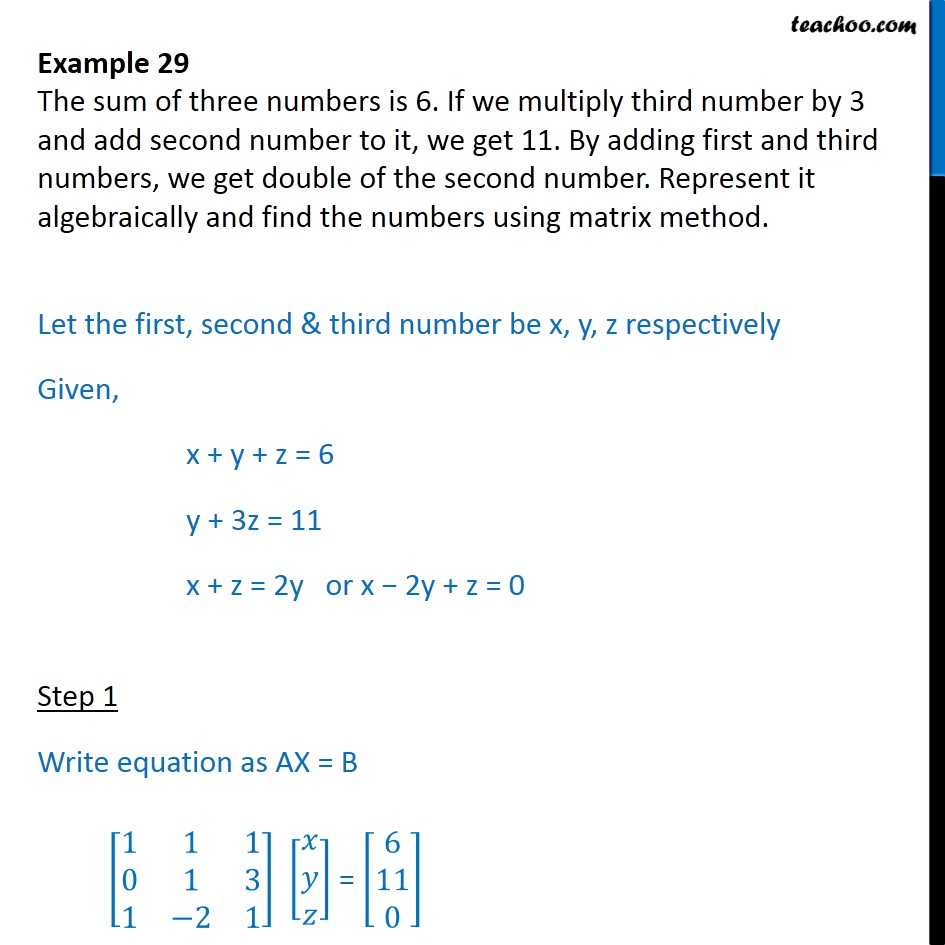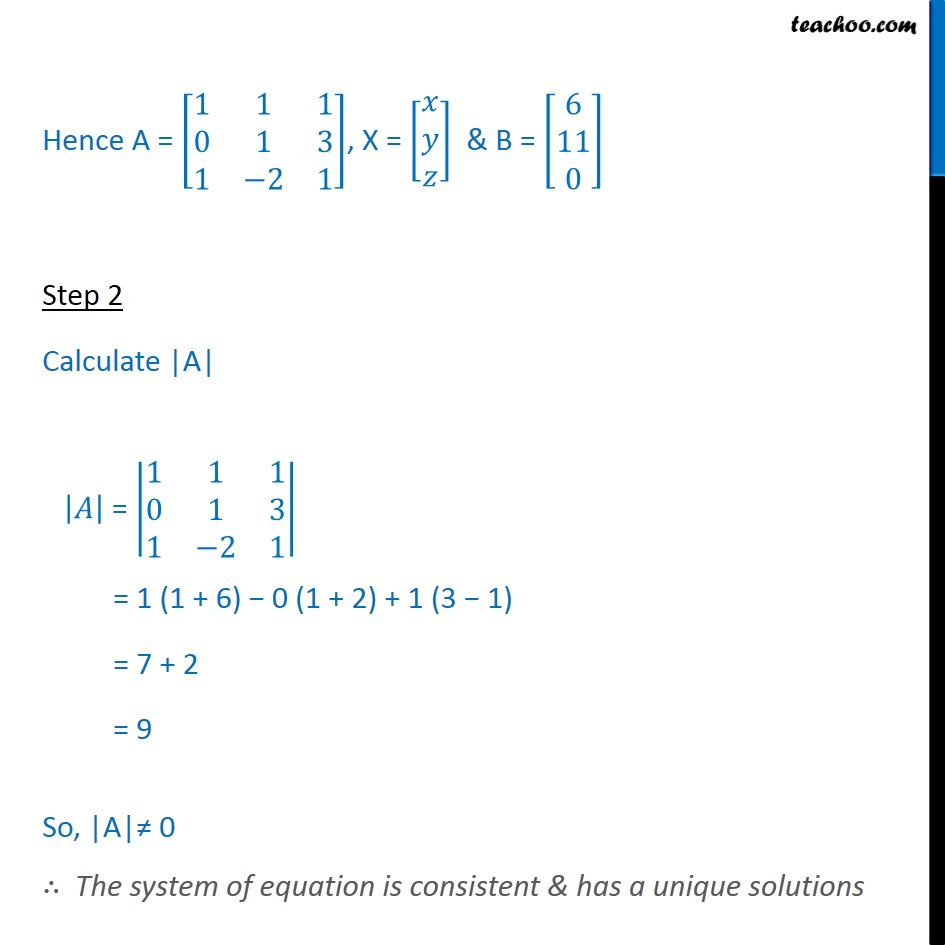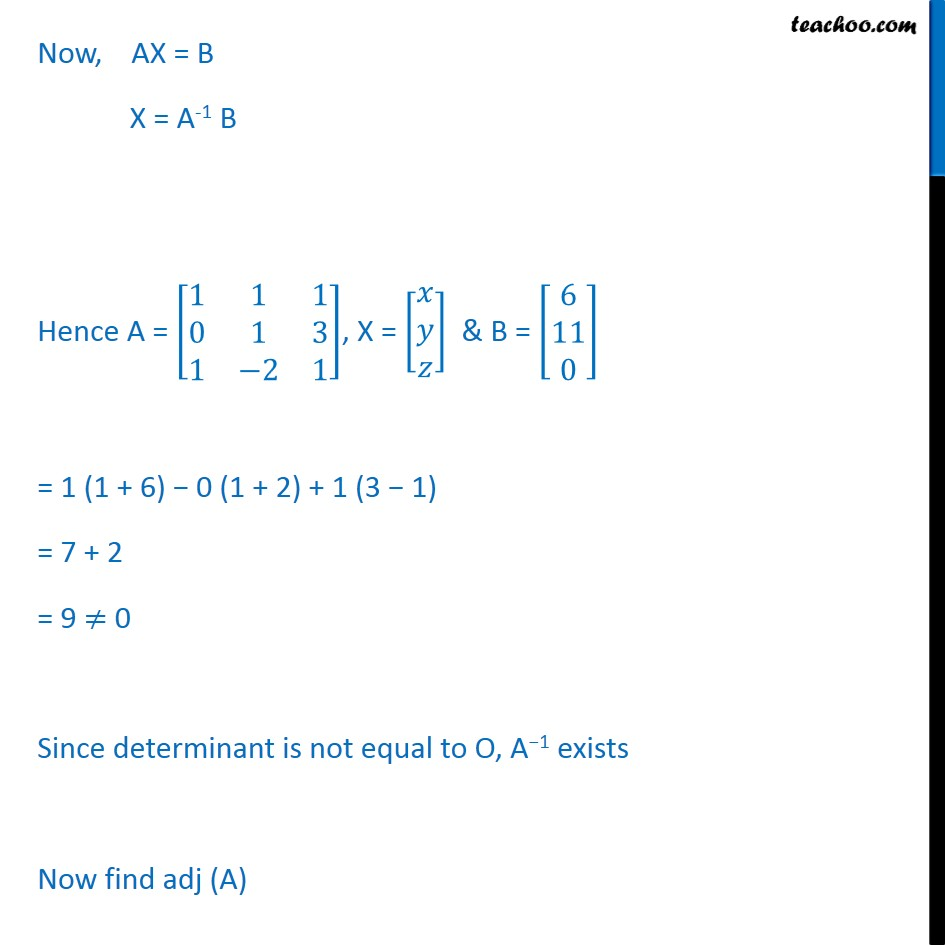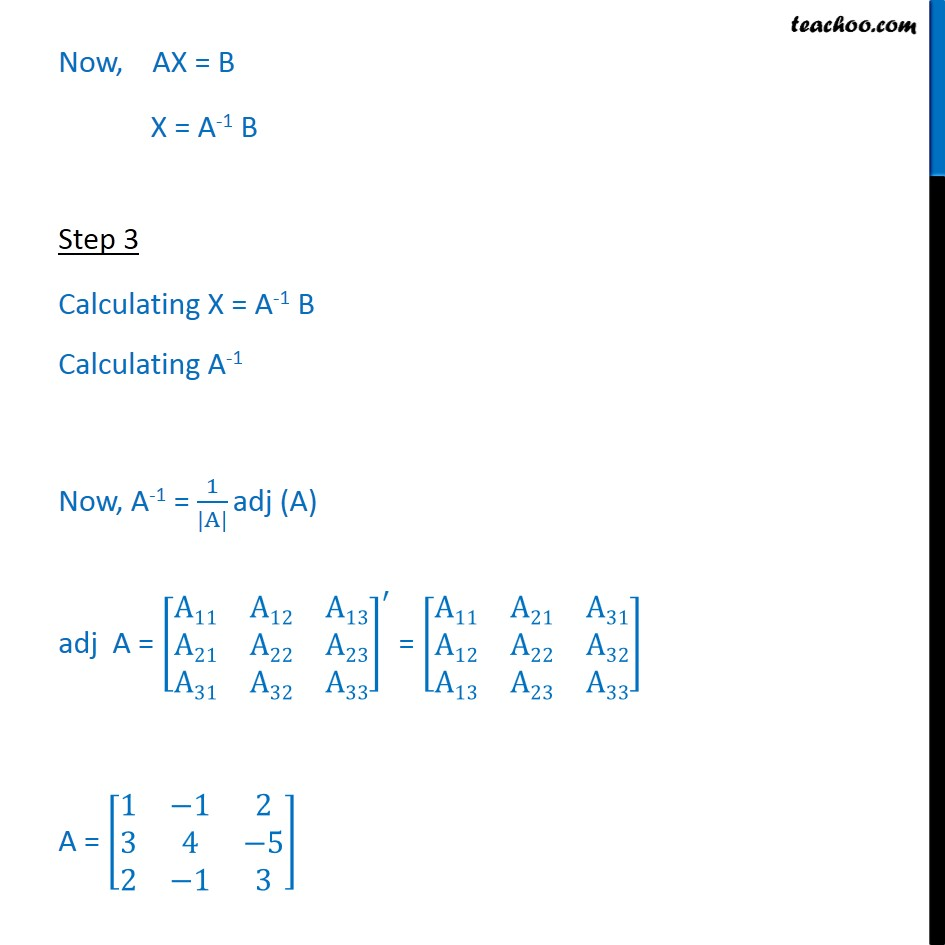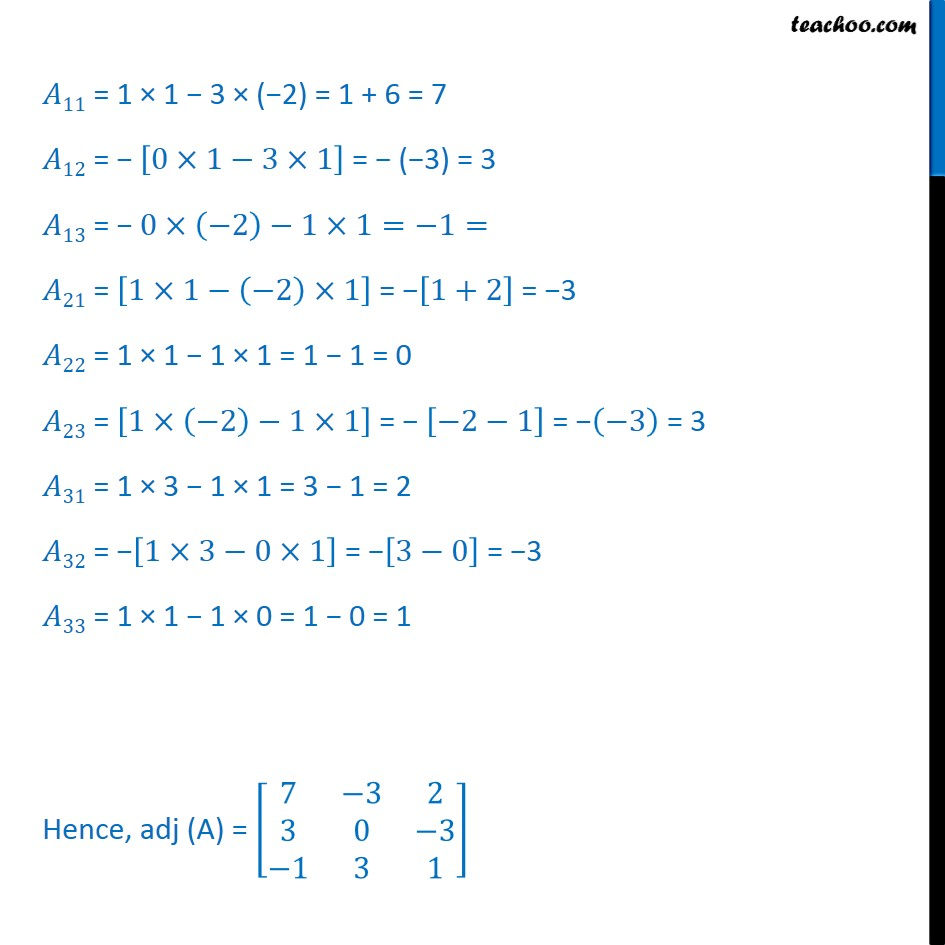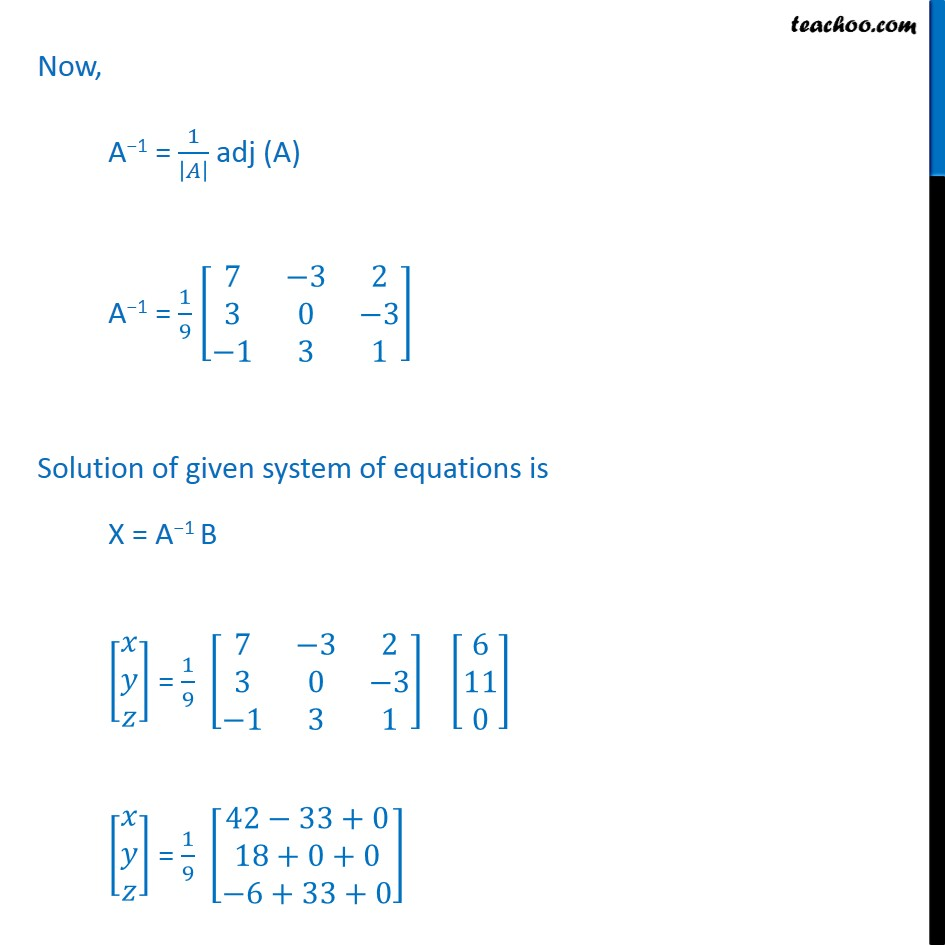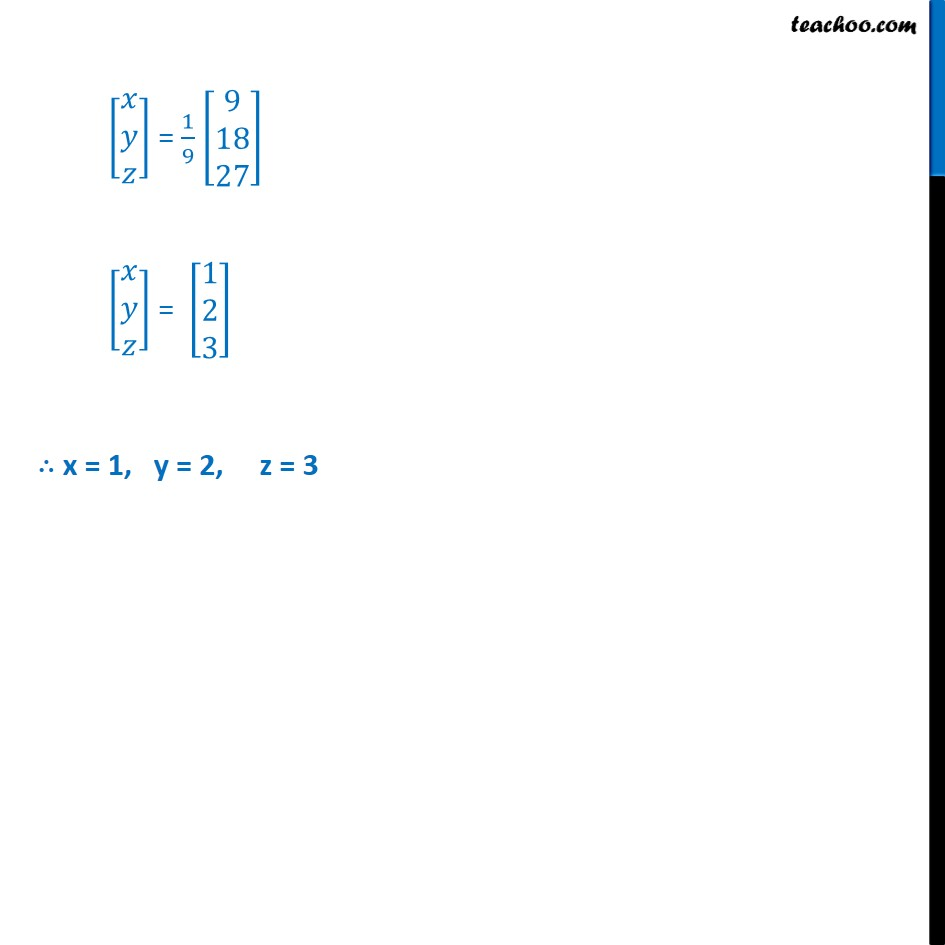Learn in your speed, with individual attention - Teachoo Maths 1-on-1 Class

### Transcript

Example 18 The sum of three numbers is 6. If we multiply third number by 3 and add second number to it, we get 11. By adding first and third numbers, we get double of the second number. Represent it algebraically and find the numbers using matrix method. Let the first, second & third number be x, y, z respectively Given, x + y + z = 6 y + 3z = 11 x + z = 2y or x 2y + z = 0 Step 1 Write equation as AX = B 1 1 1 0 1 3 1 2 1 = 6 11 0 Hence A = 1 1 1 0 1 3 1 2 1 , X = & B = 6 11 0 Step 2 Calculate |A| = 1 1 1 0 1 3 1 2 1 = 1 (1 + 6) 0 (1 + 2) + 1 (3 1) = 7 + 2 = 9 So, |A| 0 The system of equation is consistent & has a unique solutions Now, AX = B X = A-1 B Hence A = 1 1 1 0 1 3 1 2 1 , X = & B = 6 11 0 = 1 (1 + 6) 0 (1 + 2) + 1 (3 1) = 7 + 2 = 9 0 Since determinant is not equal to O, A 1 exists Now find adj (A) adj (A) = 11 12 13 21 22 23 31 32 33 = 11 21 31 12 22 32 13 32 33 Now, AX = B X = A-1 B Step 3 Calculating X = A-1 B Calculating A-1 Now, A-1 = 1 |A| adj (A) adj A = A11 A12 A13 A21 A22 A23 A31 A32 A33 = A11 A21 A31 A12 A22 A32 A13 A23 A33 A = 1 1 2 3 4 5 2 1 3 11 = 1 1 3 ( 2) = 1 + 6 = 7 12 = 0 1 3 1 = ( 3) = 3 13 = 0 2 1 1= 1= 21 = 1 1 2 1 = 1+2 = 3 22 = 1 1 1 1 = 1 1 = 0 23 = 1 2 1 1 = 2 1 = 3 = 3 31 = 1 3 1 1 = 3 1 = 2 32 = 1 3 0 1 = 3 0 = 3 33 = 1 1 1 0 = 1 0 = 1 Hence, adj (A) = 7 3 2 3 0 3 1 3 1 Now, A 1 = 1 adj (A) A 1 = 1 9 7 3 2 3 0 3 1 3 1 Solution of given system of equations is X = A 1 B = 1 9 7 3 2 3 0 3 1 3 1 6 11 0 = 1 9 42 33+0 18+0+0 6+33+0 = 1 9 9 18 27 = 1 2 3 x = 1, y = 2, z = 3